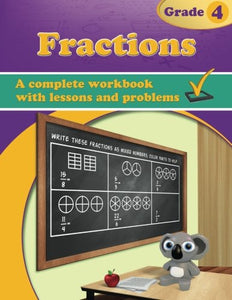Fractions, Grade 4 Workbook

• Publish Date: 2016-02-02
• Binding: Paperback
• Author: Maria Miller
Regular price \$33.43 Sale price \$18.49

Attention:For textbook, access codes and supplements are not guaranteed with used items.

Fractions, Grade 4 Workbook deals with mixed numbers, adding and subtracting like fractions and mixed numbers with like fractional parts (the denominators are the same), equivalent fractions, comparing fractions, and multiplying a fraction by a whole number.

These lessons are important because they are the basis for understanding decimal numbers. Decimals are just another way of writing fractions with denominators of 10, 100, 1,000 etc.

The topics in this chapter are studied with the help of visual models in order to emphasize the concepts.